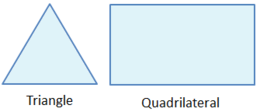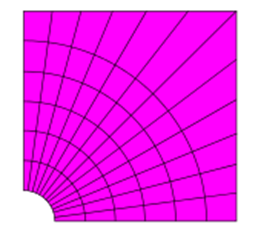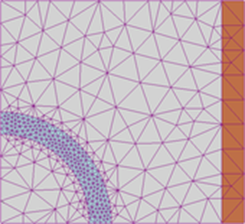# Basics of Meshing in CFD

Discretizing a domain into small elements or cells is known as meshing. Meshing is a very important part of numerical analysis. If the number of cells in a meshed domain are high the accuracy of analysis will be greater. Mesh is kept fine in the areas where capturing the physics of phenomenon is important. Mesh independence is one of the most important step in meshing. Mesh Independence is achieved by refining the mesh till the value of required variable becomes constant. Mesh refining requires high computational capabilities. Mesh can be classified into different types based on the uniformity and shape. Definitions of some elementary terms of meshing are given below (D. J. 1996).

Node: Point where two or more edges meet.

Edge: Boundary of a face

Face: Boundary of a cell

Cell: Control volume into which the domain is discretized.

Zone: Grouping of nodes faces and cells

Material data and source terms are assigned to cell zone whereas boundary conditions are applied on face zones.

# Order of Meshing:

Mesh is generated in the order given below.

Edge mesh

Boundary mesh

Face mesh

Volume mesh

# Mesh Independence:

Mesh independence is performed to see whether the size of mesh affects the solution or not. In this process, an initial mesh is generated and results are analysed for the conversion. If the conversion is not achieved, mesh is regenerated and checked to accomplish convergence. When the solution converges, mesh is refined (No of cells or elements are increased) to obtain better results. A residual limit can be set based on the problem to check for the error. With each refinement, results are obtained and compared to the results obtained with the previous mesh. If the results of two consecutive meshes are not same, mesh is refined again and again till the results become same. When the results for the two consecutive refinements are same, the previous mesh is believed to be generating accurate results.

# TYPES of Meshing Based on Cell shape and Dimension:

A mesh can be two or three dimensional (D. J. 1996)

## 1) Two Dimensional Mesh:

Two dimensional mesh can comprise of triangular or quadrilateral elements.

## Triangular mesh:

In this type of mesh, cells are of triangular shape. Comparatively a very small amount of effort and time is usually required to generate this type of mesh. Triangular mesh is usually used in the domain areas where the physics of the problem is not very important.

In this type of mesh, cells are in rectangular shape. This shape is common in two dimensional structured mesh.Figure 9 types of 2D mesh

## 2) Three Dimension

In three dimensional meshing, a cell can have a shape of quadrilateral pyramid, tetrahedron, hexahedron or triangular prism. All of them have quadrilateral and triangular faces.

Two dimensional extruded models can be represented completely by the hexahedron and prisms as extruded quadrilaterals and triangles.

In three dimensional meshing, quadrilateral faces might not be impeccably planar. A thin tetrahedron which is shared by two elements can be thought of a non-planar quadrilateral face.

## Tetrahedron:

In geometry, a tetrahedron also known as a triangular pyramid, consists of four triangular faces, four vertices, four sides and six edges. This form of mesh can be auto-generated

## Pyramid

This shape have four triangular faces, one quadrilateral face, eight edges and five vertices. They are utilized as transition elements between triangular and square elements. They are also used in hybrid grids.

## Triangular prism:

This type of cell have six vertices and nine edges bounded by three quadrilateral faces and two triangular faces. It is very effective in resolving boundary layer.

## Hexahedron

This type of cell have eight vertices and twelve edges bounded by six quadrilateral faces. Hexahedron mesh is considered best among all and it give the solution with highest accuracy.

The triangular prism and pyramid zones can be considered computationally as degenerate hexahedrons, wherein some edges were decreased to 0. Other degenerate sorts of a hexahedron may also be represented.

It is a three dimensional element which can have any number of edges, faces and vertices. Large number of calculations are performed due to large number of neighbouring cells. This mesh is created to obtain more accuracy in calculations.

Figure 10 types of mesh

## Uniform and Non Uniform Meshing:

In a uniform mesh, shape of cells throughout the domain is same whereas non-uniform mesh uses a blend of different mesh shapes. All the numerical approaches are designed to produce as small amount of errors as possible. These errors arises due to non-linear behaviour of the solution and large gradients of the physical properties in some places of the domain. This means that we need to handle small large-scale physical phenomena at the same time and these different phenomena can have a strong coupling with each other (D. J. 1996).

## Grids:

The grid labels the elements or cells on which the flow is being solved.

Represent the domain in a discrete manner

Elements are grouped into boundary zones where boundary conditions has to be applied.

The mesh affects the rate of convergence, computational time and accuracy of the solution.

Following parameters of the mesh are very important to obtain an accurate solution i.e. adjacent cellular extent/duration ratios, grid density, Tetrahedral vs. Hexahedral, skewness, mesh refinement thru adaption and Boundary/Inflation layer mesh.

## Structured grids:

A structural grid spreads over a domain in a regular manner. In two dimensional meshing, structural grids have quadrilateral elements whereas in three dimensional structural meshing the elements are of hexahedron type. Structural gird is extremely space efficient. They display better solution convergence and generate accurate numerical results.

## Unstructured grids:

Unstructured grids spread over a domain in an irregular manner. This type of mesh can be generated using any type of elements. The values at the nodes of unstructured mesh are very difficult to be represented in two or three dimensional arrays. Comparatively, an unstructured mesh is less space efficient. In two dimensional unstructured mesh, triangular shaped elements are used whereas in three dimensional unstructured mesh the elements are of tetrahedral shapeFigure 11  structured and non-structured Grid

## Hybrid grids:

A hybrid mesh have both structured and unstructured mesh patches spread over the entire domain in a well-organized way. In the hybrid mode of meshing, structured grid is generated in the plain areas while unstructured meshing is generated in the areas where geometry is complex